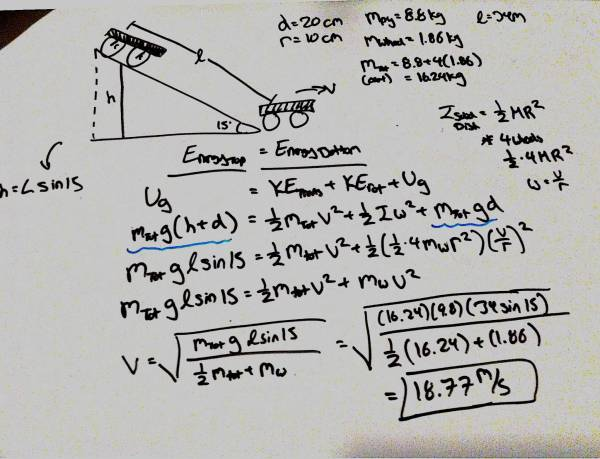# Conservation of Angular Momentum of Cart Problem

• carhartt
In summary, the child built a simple cart consisting of a 0.60 m by 1.20 m sheet of plywood and four wheels, each 20.0 cm in diameter and with a mass of 1.86 kg. The cart was released from the top of a 15° incline that was 34 m long. The speed at the bottom was found to be 18.8 m/s.

## Homework Statement

A child builds a simple cart consisting of a 0.60 m by 1.20 m sheet of plywood of mass 8.8 kg and four wheels, each 20.0 cm in diameter and with a mass of 1.86 kg. It is released from the top of a 15° incline that is 34 m long. Find the speed at the bottom. Assume that the wheels roll along the incline without slipping and that friction between the wheels and their axles can be neglected. (You may assume that the center of mass of the cart starts at very top of the incline.)

Diameter = 20 cm, Massplywood = 8.8kg, Masswheel = 1.86 kg
Length of incline = 34 m Masstotal = 16.24 kg

## Homework Equations

Energybefore = Energyafter

KEtranslational = 1/2mtotalv2
KErotational = 1/2 I ω2
Isolid disk = 1/2 M R2
Ug = mgh
v = rω

## The Attempt at a Solution

Attached is a copy of my work at the solution.
1. After drawing a sketch of my problem I set my energies from the top of the incline equal to the energies when at the bottom.
2. The only energies acting in "before" is gravitational potential energy Ug = mtotalgh. I can find the high using trig h = Lsin(15). Note the gravitational potential energy of the carts center of mass mtotalgd will cancel out on the "left" side of the equation.
3. The energies acting in "after" is the translational KE, rotational KE, and gravitational potential energy (that cancels out mentioned in 2).
- KEt = 1/2mtotalv2
- KEr = 1/2(1/2*4MwheelR2)(v/r)2
++ the moment of inertia for a solid disk is I = 1/2 M R2 but there are 4 wheels hence multiplied by 4. Also v = rω, substituting (v/r) for ω.
4. Simplified and solve for velocity.

I am doing this homework assignment on webssign and 18.8 m/s 18.77 m/s, whichever, does not seem to be correct.Operator error: Make sure that your calculator is set to use degrees rather than radians.

gneill said:
Operator error: Make sure that your calculator is set to use degrees rather than radians.

Of course, that was it. Thank you.